# Heat Transfer by Natural Convection (Part - 1) Chemical Engineering Notes | EduRev

## Chemical Engineering : Heat Transfer by Natural Convection (Part - 1) Chemical Engineering Notes | EduRev

The document Heat Transfer by Natural Convection (Part - 1) Chemical Engineering Notes | EduRev is a part of the Chemical Engineering Course Heat Transfer for Engg..
All you need of Chemical Engineering at this link: Chemical Engineering

Heat transfer by Natural Convection, Heat Transfer

5.1 Introduction

In the previous chapter, we have discussed about the forced convective heat transfer when the fluid motion relative to the solid surface was caused by an external input of work by means of pump, fan, blower, stirrer, etc. However, in this chapter we will discuss about the natural or free convection. In natural convection, the fluid velocity far from the solid body will be zero. However, near the solid body there will be some fluid motion if the body is at a temperature different from that of the free fluid. In this situation there will be a density difference between the fluid near the solid surface and that far away from the system. There will be a positive or negative buoyancy force due to this density difference. Hot surface will create positive buoyancy force whereas the cold surface will create the negative buoyancy force. Therefore, buoyancy force will be the driving force which produce and maintain the free convective process. Figure 5.1 shows the natural convective process for a hot and cold vertical surface.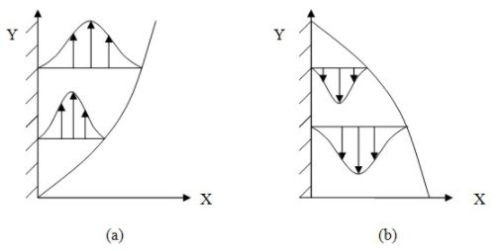Fig.5.1: Free convection boundary layer for vertical (a) hot surface and (b) cold surface

Consider a vertical flat plate with contact of a fluid (say liquid) on one side of the plate. Now assume that we raise the temperature of the plate to Ts, a natural convective boundary layer forms as shown in fig. 5.2. The velocity profile in this boundary layer is slightly different as compared to forced convection boundary layer. At the wall the velocity is zero because of no slip condition. The velocity increases to maximum and then reduces to zero at the end of the boundary layer because the fluid is at rest in the bulk. Initially the laminar flow is achieved in the boundary layer, but at some distance from the leading edge, depending on the fluid properties and the temperature difference between plate and bulk fluid, turbulent eddies are found thus laminar to transition region comes. On further away from the leading edge the boundary layer may become turbulent and the boundary layer instability comes in to picture. Instability of the boundary layer is quite complex and does not fall into the scope of this study material.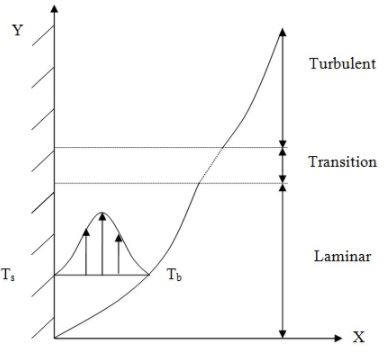Fig. 5.2: Boundary layer on a hot vertical flat plate (Ts: surface temperature; Tb: bulk fluid temperature)

It has been found over the years that the average Nusselt number (or the average heat transfer coefficient) for convective heat transfer can be represented by the following functional dependence (say viscous flow past a hard body).

Nu = f(Re,Gr,Ec,Pr)          (5.1)

The Reynolds number (Re) is the ratio of inertia forces in the fluid to the viscous forces. The Grashof number (Gr) is the ratio of buoyant forces to the viscous forces. The Eckert number (Ec) is a measure of the thermal equivalent of kinetic energy of the flow to the imposed temperature differences. The Eckert number arises due to the inclusion of viscous dissipation. Thus Ec is absent where dissipation is neglected. The Prandtl number, Pr, is the ratio of the momentum diffusivity (kinematic viscosity) to the thermal diffusivity. In other words, Prandtl number is a measure of the relative magnitude of the diffusion of momentum, through viscosity, and the diffusion of heat through conduction, in the fluid.

In case of perfect natural-convection and in absence of heat dissipation, the eq. 5.1 reduces to,

Nu = f(Gr,Pr)              (5.2)

It is to be noted that in case of perfect natural convection, the main fluid stream is absent, thus Reynolds number is no longer significant.

The dimensionless numbers involved in eq. 5.2 evaluated at the average film temperature,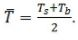It can be easily found that in case of the forced convection and in absence of heat dissipation the function for average heat transfer will be,

Nu = f(Re,Pr)                     (5.3)

On comparing eq. 5.2 and 5.3, one can see that the Grashof number will perform for free convection in a same way as the Reynolds number for forced convection.

Another parameter, the Rayleigh number is also used for perfect natural-convection is defined as,

Ra = Gr . Pr               (5.4)

Thus the functional relation is eq. 5.2 can be written as,

Nu = f(Ra,Pr)                   (5.5)

As discussed earlier that all free convection flows are not limited to laminar flow. If instability occurs, the problem becomes complex. A general rule one may expect that transition will occur for critical Rayleigh number of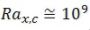(5.6)

The Grashof number is defined as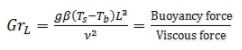where,

 g = acceleration due to gravity β = coefficient of volume expansion = Ts = surface temperature Tb = bulk fluid temperature L = characteristic lenght v = Momentum diffusivity (kinematic viscosity)
Offer running on EduRev: Apply code STAYHOME200 to get INR 200 off on our premium plan EduRev Infinity!

## Heat Transfer for Engg.

46 videos|60 docs|59 tests

,

,

,

,

,

,

,

,

,

,

,

,

,

,

,

,

,

,

,

,

,

;